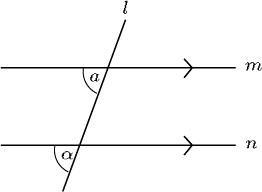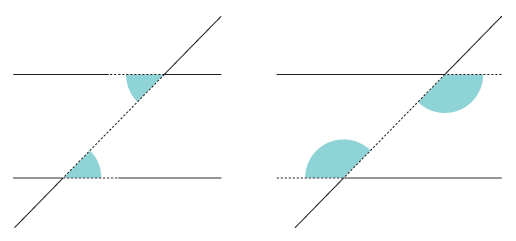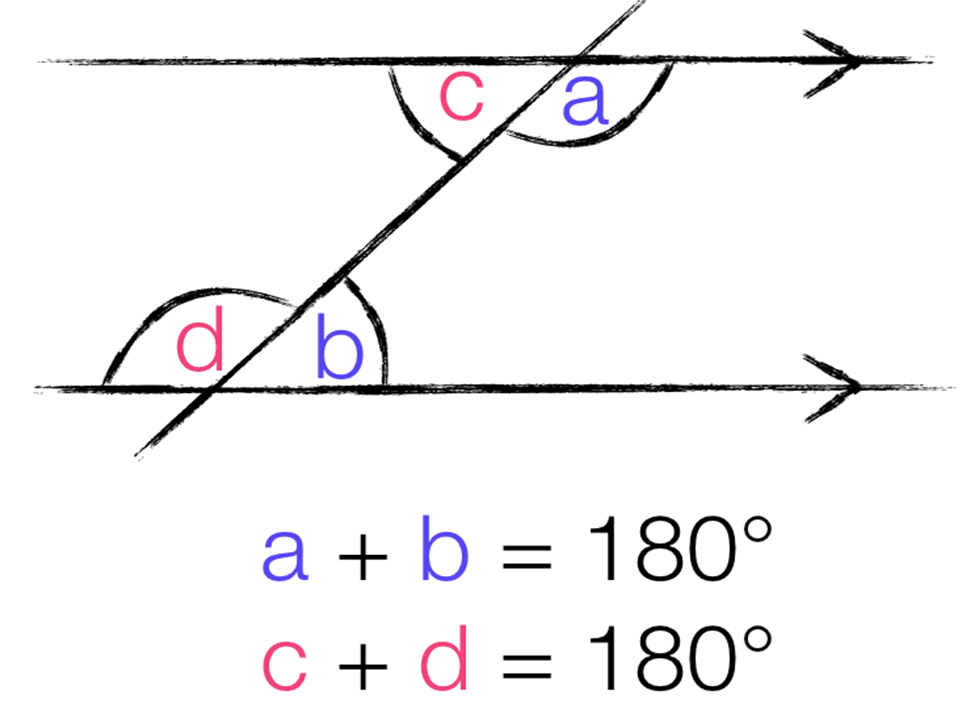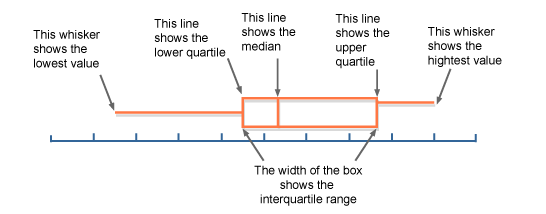# Maths

?

Maths

Angles:

• Sum of the interior angles: 180(n-2)

• The exterior angles in a polygon add up to 360 degrees

Corresponding anglesAlternate anglesInterior anglesTrigonometry for Right-angled Triangles:SOH CAH TOA

SOH stands for sine=opposite/hypotenuse

1. Label Sides

1. Side opposite to unknown angle should be labeled as opposite

2. The side next to unidentified angle should be labeled as adjacent

3. Longest side of triangle should be labeled as hypotenuse

Sine, cosine, tangent - Side lengths of a triangle

Sine-1, cosine-1, tangent-1 - Angles of a triangle

Box Plots:Cumulative Frequency Diagrams:

Cumulative frequency: Adding up the frequencies as you go